##### Pre-Calculus All-in-One For Dummies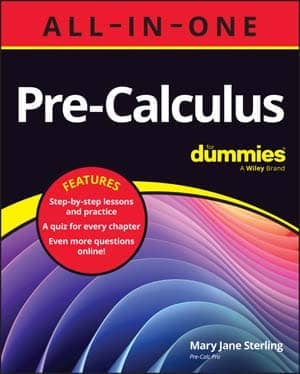Remembering the properties of numbers is important because you use them consistently in pre-calculus. The properties aren’t often used by name in pre-calculus, but you’re supposed to know when you need to utilize them. The following list presents the properties of numbers:

• Reflexive property. a = a. For example, 10 = 10.

• Symmetric property. If a = b, then b = a. For example, if 5 + 3 = 8, then 8 = 5 + 3.

• Transitive property. If a = b and b = c, then a = c. For example, if 5 + 3 = 8 and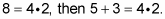• Commutative property of addition. a + b = b + a. For example, 2 + 3 = 3 + 2.

• Commutative property of multiplication.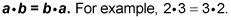• Associative property of addition. (a + b) + c = a + (b + c). For example, (2 + 3) + 4 = 2 + (3 + 4).

• Associative property of multiplication.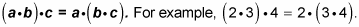• Additive identity. a + 0 = a. For example, –3 + 0 = –3.

• Multiplicative identity.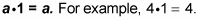• Additive inverse property. a + (–a) = 0. For example, 2 + (–2) = 0.

• Multiplicative inverse property.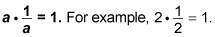• Distributive property.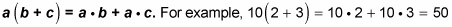• Multiplicative property of zero.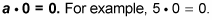• Zero-product property.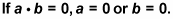For example, if x(x + 2) = 0, then x = 0 or x + 2 = 0.

If you’re trying to perform an operation that isn’t on the previous list, then the operation probably isn’t correct. After all, algebra has been around since 1600 BC, and if a property exists, someone has probably already discovered it. For example, it may look inviting to say that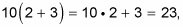but that’s incorrect. The correct answer is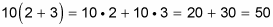Knowing what you can’t do is just as important as knowing what you can do.